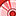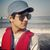# New to Qlik Sense

If you’re new to Qlik Sense, start with this Discussion Board and get up-to-speed quickly.

Announcements
Read about the latest Qlik Community enhancements on the Community News blog!
cancel
Showing results for
Did you mean:Contributor III

## COUNT with multiple conditions

Hi All,

I get stuck with below expression.

What i am trying to do is to perform a distinst count for style number, if

Condition 1 : [Yearly_Erd In Dc Date] is = 2019 (AND)

Condition 2: [Quantity] is not equal to 0

Given the result will need to include the filter which user apply.

When i use the formula below, i am not able to get my result, it just give me "-" from the result.

=Count({\$<[Yearly_Erd In Dc Date]={'\$(=Year(Today()))'}>}, <Quantity = - {0}>} distinct [Style Number])

1 Solution

Accepted SolutionsSpecialist III

=Count({\$<[Yearly_Erd In Dc Date]={'\$(=Year(Today()))'}>}, Quantity -=  {0}>} distinct [Style Number])

try

Channa
6 RepliesSpecialist III

=Count({\$<[Yearly_Erd In Dc Date]={'\$(=Year(Today()))'}>}, Quantity -=  {0}>} distinct [Style Number])

try

ChannaSpecialist

HI,

Try this :
=Count(distinct{<[Yearly_Erd In Dc Date]={"=\$(=year(today()))"},Quantity -={0}>}[Style Number])

RegardsPartner

Hi Bbastro,

There is a problem of your column [Yearly_Erd In Dc Date],

because you are using [Yearly_Erd In Dc Date] = 2019, so you need to make sure that the value of [Yearly_Erd In Dc Date] is not date type, it should be "year" type, like 2019, 2018, 2017....

And if it's not "year" type, you may try to use below expression:

`=Count({<[Yearly_Erd In Dc Date] = {">=\$(='2019-01-01')<=\$(='2019-12-31')"},Quantity -= {"0"}>}distinct [Style Number])`

Aiolos ZhaoSpecialist III

=Count(distinct{<year([Yearly_Erd In Dc Date])={"=\$(=year(today()))"},Quantity -={0}>}[Style Number])

if this dosent work

create one new column in script

year([Yearly_Erd In Dc Date]) as Year

and use below

=Count(distinct{<year={"=\$(=year(today()))"},Quantity -={0}>}[Style Number])

ChannaContributor III
Author

The value of [Yearly_Erd In Dc Date] is not a date type, it show like 2018, 2019...etc.Specialist III

=Count(distinct{<[Yearly_Erd In Dc Date]={'\$(=Max([Yearly_Erd In Dc Date]))'},Quantity -={0}>}[Style Number])

use this no need to use today()

ChannaTags
Community Browser